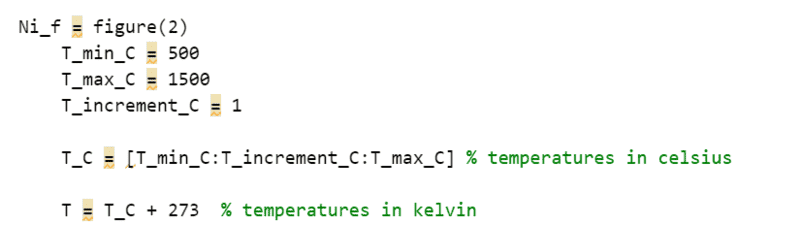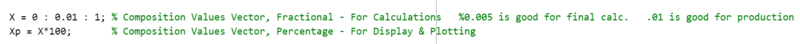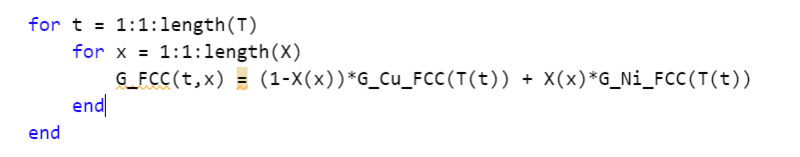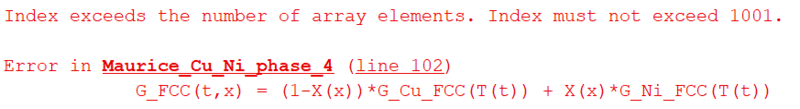# Populating a 1001x101 2D array in MATLAB

• Sai Maurice
In summary, the method I employed was based on a nested loop. I ran into two issues with this approach: 1) the code took way too long to run, easily going for over 7 minutes, and 2) in the end, it didn't even completely work, due to the "index exceeding the array length". This confuses me.f

#### Sai Maurice

Homework Statement
The goal is to create a 2d array of Gibbs free energy of a copper-nickel solution as a function of temperature and composition in percent nickel, G(T,X). Additional requirements are based on time, where running of this code cannot take more than a few seconds.
Relevant Equations
G(T,X) = (1-X)*Gcu(T) + X*Gni(T)
Where:
T = a 1001 member array of temperatures in kelvin
X = a 101 member array of values for percent composition of nickel from 0 to 1
Gcu(T) = known values for the Gibbs free energy of copper at a given temperature T
Gni(T) = known values for the Gibbs free energy of nickel at a given temperature T
The method I employed was based on a nested loop. I ran into two issues with this approach
1. The code took way too long to run, easily going for over 7 minutes.
2. In the end, it didn't even completely work, due to the "index exceeding the array length". This confuses me
For the relevant sections, my code looks like the following:How do I populate this 1001x101 2d array without relying on loops? Why am I exceeding the array length? Thank you for the feedback

Caveat: I'm not at all an expert in Matlab...
Regarding your two questions, 1) I don't think there's an alternative to using nested loops to initialize your 2D matrix. As far as the time taken, >7 minutes, that seems to be way too long for a matrix with only about 100,000 elements. Seeing the whole script might offer a clue as to why it takes so long to run.

2) As for the index error, I don't see anything that sticks out in the code images you posted. It would be helpful to post the whole script. Please do so using code tags, as in the example below.
[code=matlab]for t = 1:length(T)
<etc.>
end[/code]
I'm reasonably sure you don't need to include the increment if it is 1.
Is there somewhere in your code where you declare/initialize the matrix G_FCC? Such as for example G_FCC = zeros(1001, 101).
If you have declared it to have 101 rows and 1001 columns, that would cause a problem.

First get the code working on something small like a 4 x 8 array.

Several things.
1) The size of G_FCC increases with every loop update, forcing Matlab to continually redefine the memory allocated to this array. This is extremely slow. Place a command like
G_FCC = zeros(1001,101);
before your loops to allocate the array memory once. It will run more quickly.
2) Matlab should have given you a warning about #1, by the way--hover your cursor over the short orange dashes in the right-hand border to read the warnings. For many issues, you can click on "Fix" and Matlab will correct it for you. A red line in the border alerts you to a syntax errror that must be corrected before your script will run.
3) Matlab (Matrixlaboratory) is a linear algebra engine, so array and matrix operations will always be vastly quicker than loops. Recast your problem.
You aren't showing your full code, but I'm assuming that G_Cu_FCC and G_Ni_FCC are function calls. If that is so, then you can replace your loops with this:
G_FCC = G_Cu_FCC(T.') * (1 - X) + G_Ni_FC(T.’) * X;
(You don't need to preallocate G_FCC in this case because this command defines it all at once.)
You won't believe the speed-up this gives.

Last edited:
•Mark44